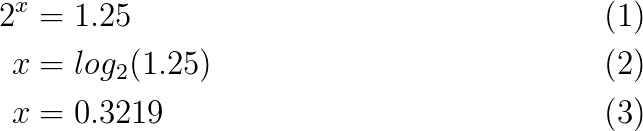Show Question
Math and science::INF ML AI

# Shannon information content

For an ensemble, $$X = (x, A_x, P_x)$$, the Shannon information content of an event, $$x$$ is defined to be:

$h(x) = log_2 \frac{1}{P(x)} \\ \text{Where 'x' may be an outcome: a subset of } A_x$

It is measured in bits.

The information content of an event/information is equivalent to the factor (represented as the exponent of 2) by which the space of possible outcomes shrinks if it is to be assumed that event/information $$x$$ is true.  Can may be helpful for intuition to write $$log(\frac{1}{P(x)})$$ as $$-log(P(x))$$.

The information content comes in this form so that the combination of multiple events/information can be easily related. For example, if an event A causes the set of possible outcomes to shrink by a factor of 4, and another event, event B, causes the set of possible outcomes to shrink further by a factor of 8, then the overall factor of shinkage is 32. The information content as presented here has a multiplicative effect. If we want information content to have the property of additivity in this case, then we can represent the information content as the exponent of some base: $$c^{log_c(4) + log_c(8)} = 32$$. For the Shannon information content, the base is 2. This makes the information content 2 bits and 3 bits respectively for a total of 5 bits: $$32 = 2^2 + 2^3$$.

### Example

If in a class of 32 people it is someones birthday, and I tell you that the person whose birthday it is has blue eyes and 26 out of the 32 people have blue eyes, then the space of possible outcomes has reduced from 32 to 26. This is a factor of $$\frac{32}{26} = 1\frac{1}{4}$$.\begin{align} 2^x &= 1.25 \\ x &= log_2(1.25) \\ x &= 0.3219 \end{align}

So the Shannon information content of the information or event "the birthday person has blue eyes" is 0.3219 bits.

If instead there were only 2 people with blue eyes in the class, the space of possible outcomes reduces to 2. This is a reduction by a factor of 16. The Shannon information content is thus 4 bits.